"Few vintage tube amps have attained the gilded reverence heaped upon the Vox AC30. Certainly, the late 1950s Fender tweeds and Marshall's JTM45 and Plexi amps are equally lust-worthy in the eyes and ears of many players and collectors, but JMI's creation is often discussed with an added catch of the breath, an excited charge in the air. There are vintage tone monsters and then there's the tone goddess - the AC30." -Dave Hunter1

#### First Stage 12AX7 Preamp

The first stage preamp contains two voltage amplifiers, one for the bright inputs and one for the normal inputs, that share a 1.5kΩ cathode resistor and 25μF bypass capacitor. Only the normal channel is shown here.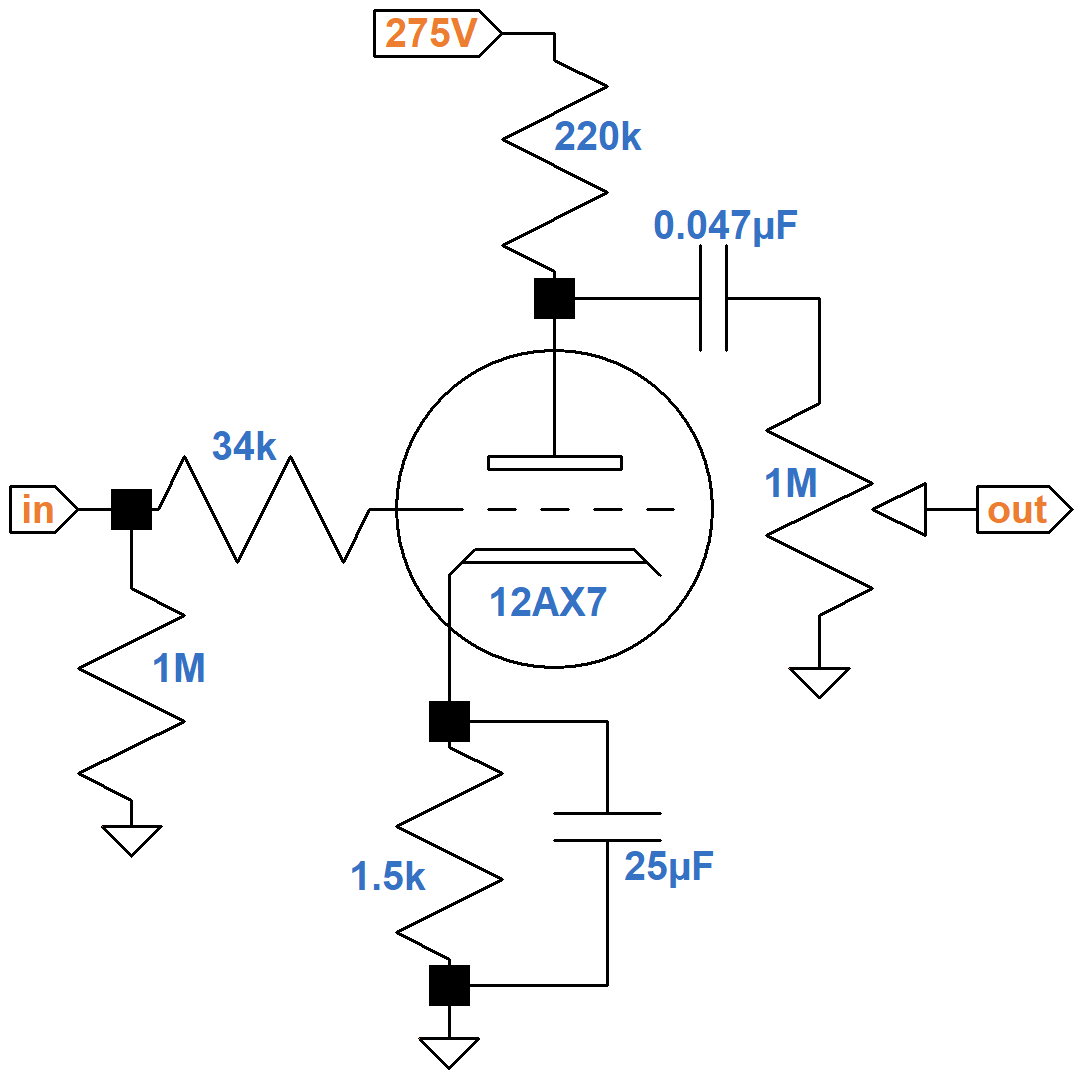Under DC conditions the cathode resistor carries the current of two triodes, so its effective resistance per triode is 3kΩ, double its actual value. For a 275V plate supply and a 220kΩ plate load resistor, the 12AX7 calculator shows that the DC grid bias is -1.6V.The AC load for each channel is a 500kΩ volume control. The calculator indicates that the DC current load on the power supply is a modest 0.5mA.

The DC grid bias is slightly cooler than neutral: 1.4V from cutoff according to the AC load line. This makes the input headroom 1.4V peak (-0.1dBV). This is well beyond the maximum output signal level from a typical pickup.New! Guitar Amplifier Electronics: Fender Deluxe - from TV front to narrow panel to brownface to blackface Reverb

According to the Preamp Gain and Output Impedance calculator, the unloaded gain and output impedance are 37.8dB and 49kΩ, respectively.According to the Coupling Capacitor calculator, the load of the 500kΩ volume control attenuates the output by 0.8dB, making the loaded gain 37dB. The large 0.047μF coupling capacitor creates a flat response - attenuation at 82Hz is less than a tenth of a decibel. Gain at 10Hz, well below audio, is down by more than decibel, which contributes to inter-stage decoupling.Guitar Amplifier Electronics: Basic Theory - master the basics of preamp, power amp, and power supply design.

#### First Stage Bright Channel

The bright channel uses a much smaller coupling capacitor and adds a bright bypass capacitor across the top of the volume control.The Bright Boost Capacitor Calculator shows the effect of the 100pF capacitor when the volume control is at 50-percent rotation (10-percent resistance).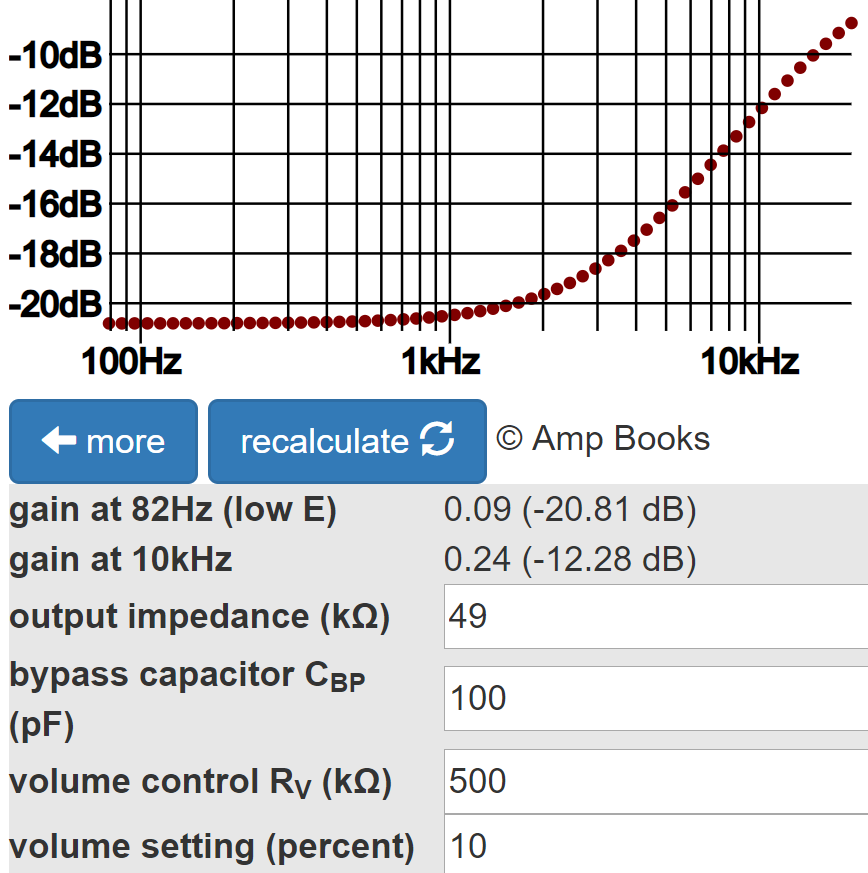Gain is up by more than 8dB at 10kHz.

The bright channel also uses a much smaller coupling capacitor for a huge amount of bass cut. According to the Coupling Capacitor calculator, gain is down by 2dB at midrange and almost 18dB at 82Hz.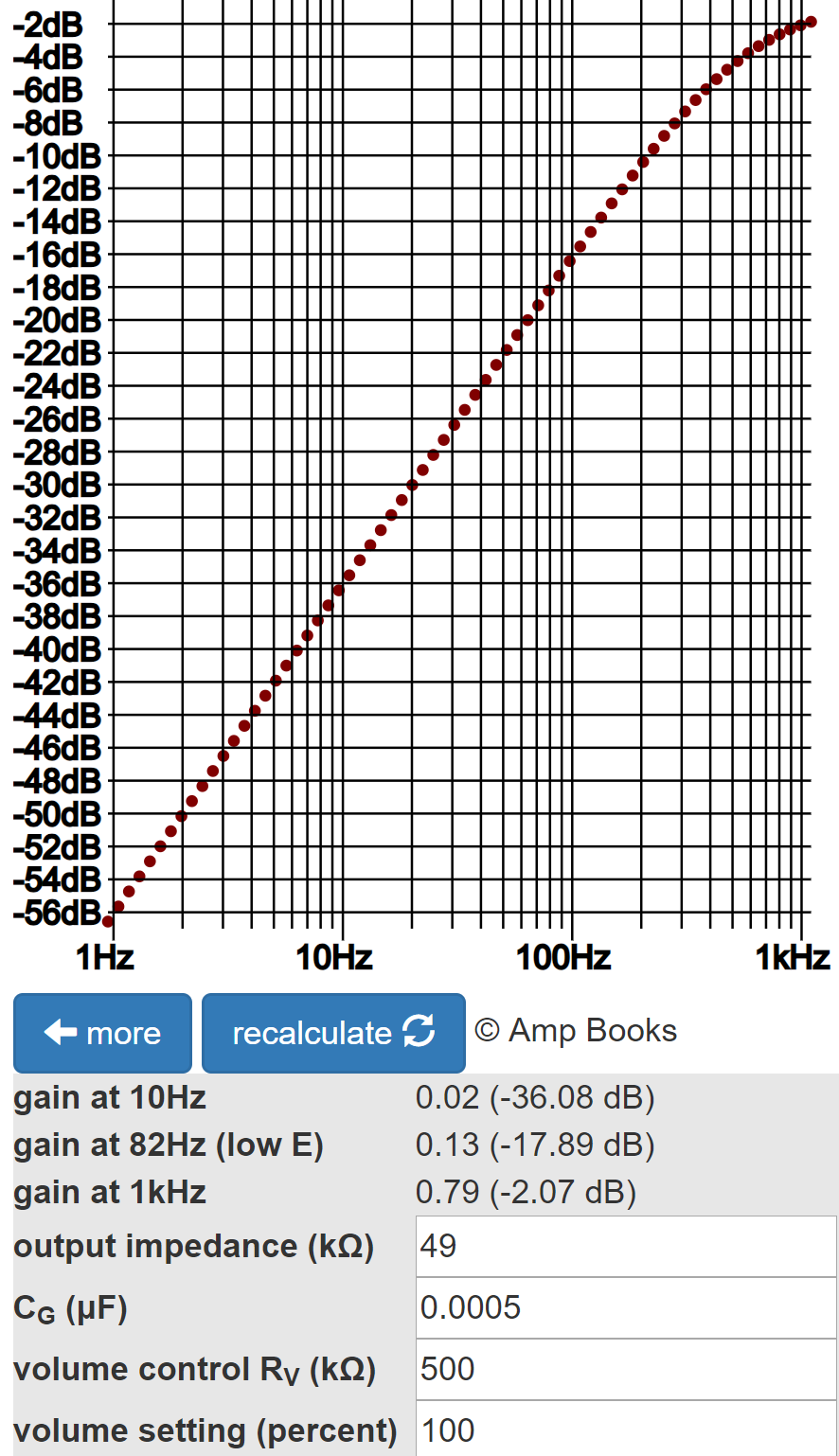Fundamentals of Guitar Amplifier System Design - design your amp using a structured, professional methodology.

#### Second Stage Voltage Amp

The cathode resistor for the second stage voltage amplifier is not bypassed by a capacitor, creating negative feedback from cathode degeneration.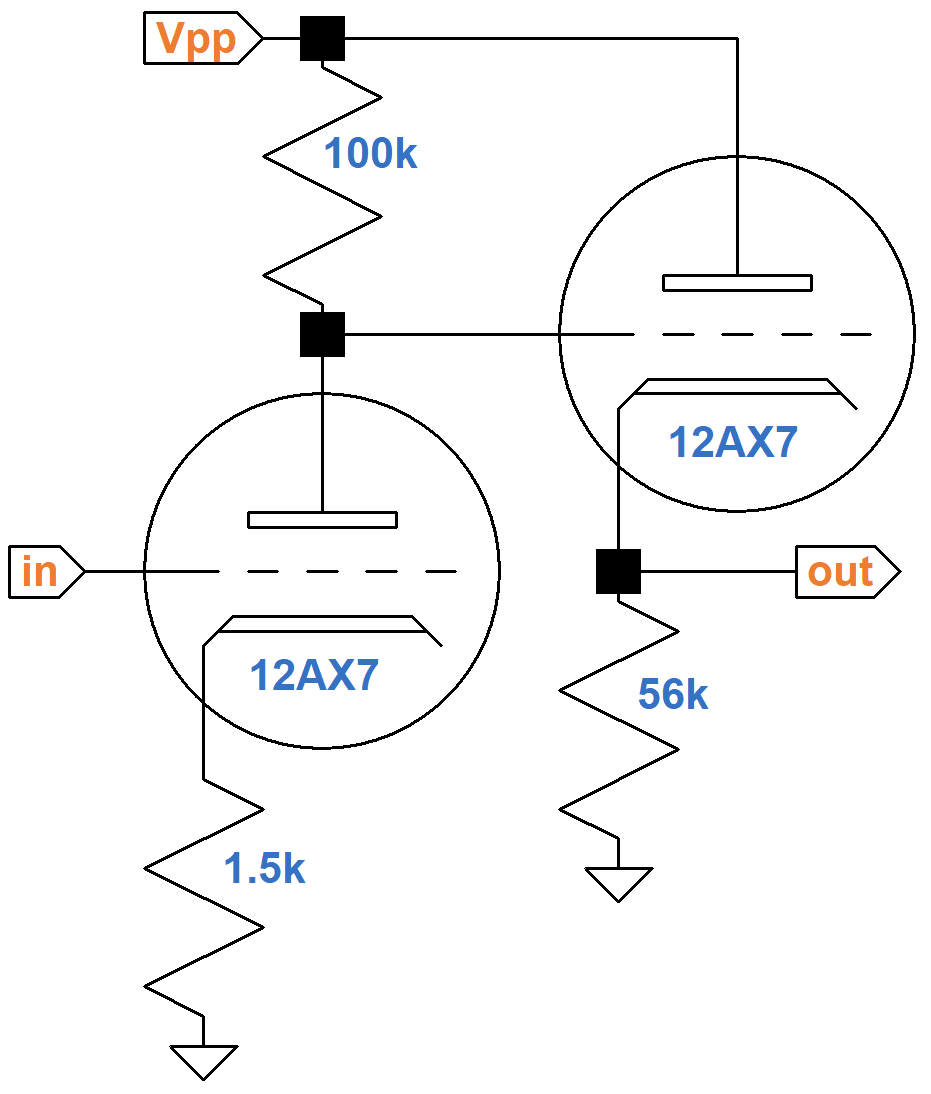This reduces gain and increases output impedance. The former is a design choice to create the amount of gain desired within the context of system input sensitivity and the stage's position in the signal chain. The latter is not an issue, because the voltage amplifier drives a light load that does not demand a lot of current. The Preamp Gain and Output Impedance calculator indicates that the stage has a gain of 30dB and an output impedance of 68kΩ.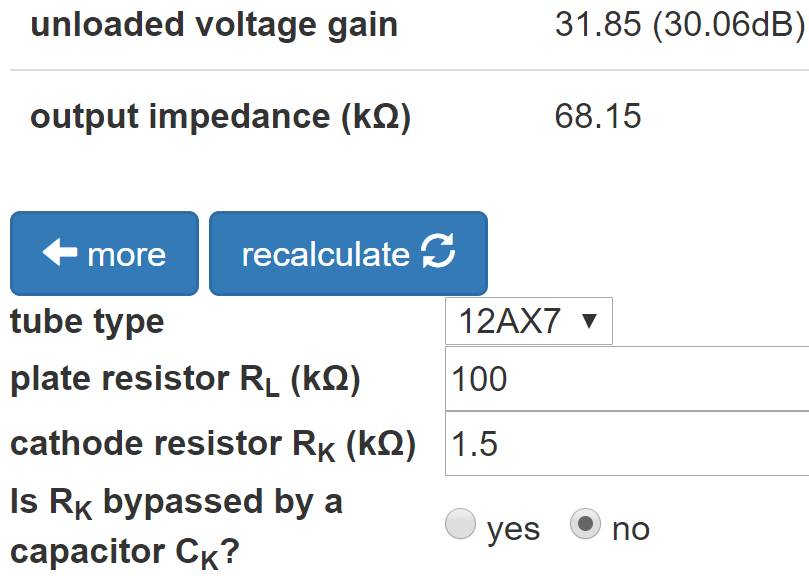According to the Cathode Follower calculator, the output impedance is only 612Ω.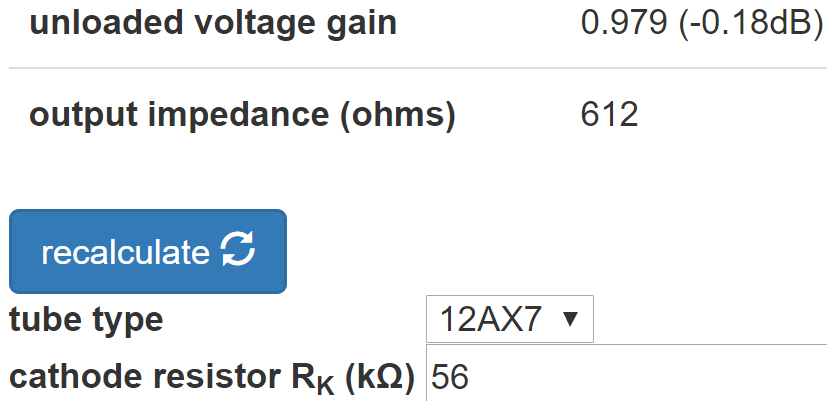In an environment of resistances measured in kiloohms, this is very low, making it ideal for driving a tone stack that demands a lot of current.Guitar Amplifier Electronics: Circuit Simulation - know your design works by measuring performance at every point in the amplifier.

#### Tone Stack

The tone stack has an architecture that is similar to the Fender Bassman, but without a middle control.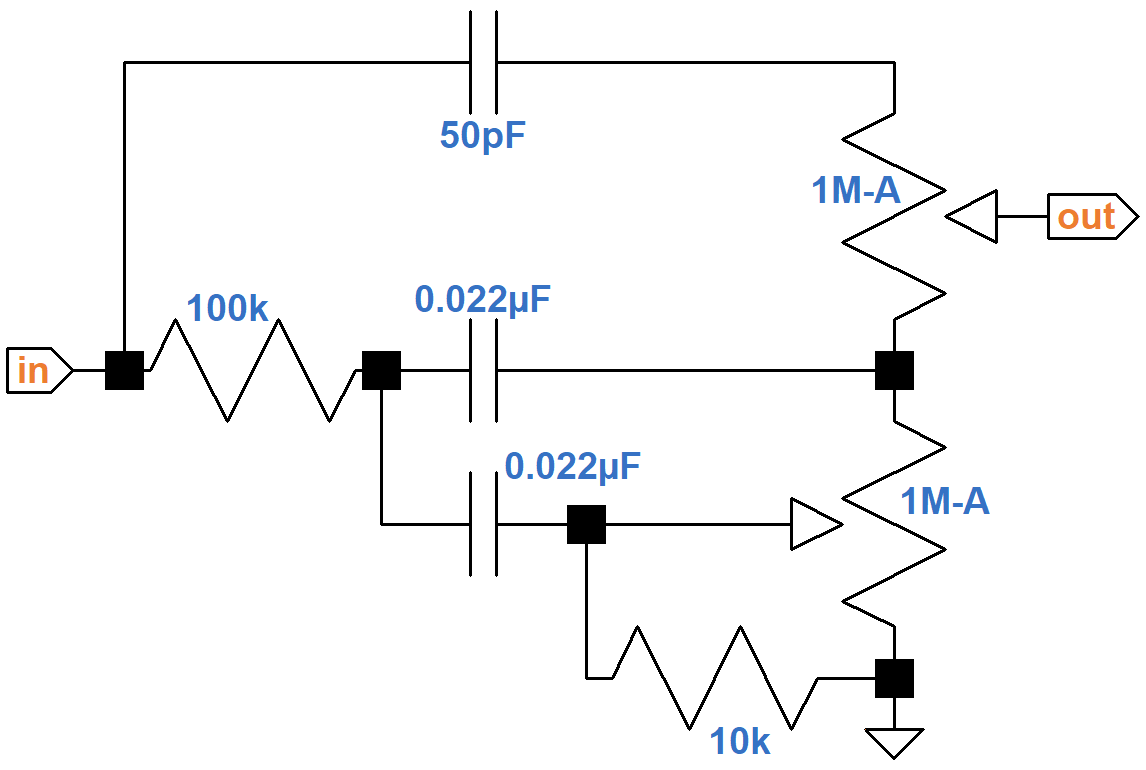Based on SPICE simulation,2 this plot of gain in dB versus frequency in hertz assumes the source impedance is 614Ω and the output load is 544kΩ, which is approximately the case when the normal channel volume control is set to maximum.Maximum scoop for the orange trace is at 480Hz. The crossover point for the other three traces is at 780Hz.

#### Long-Tailed-Pair Phase Inverter

The phase inverter is a long tailed pair in its purest form:

• The non-inverting input is connected to AC ground.
• The tail resistor is not split to provide an insertion point for negative feedback.
• In fact, there is no negative feedback in the Vox AC30!The resistors connecting the cathodes to ground carry the current of two triodes, so for the purposes of determining the DC operating point, the equivalent circuit for one triode doubles the resistor values. This puts a total of 100kΩ + 94kΩ = 194kΩ plus the bias resistor in series with the tube. According to the 12AX7 calculator, the effective 2.4kΩ bias resistor creates a DC grid bias of -1.5V.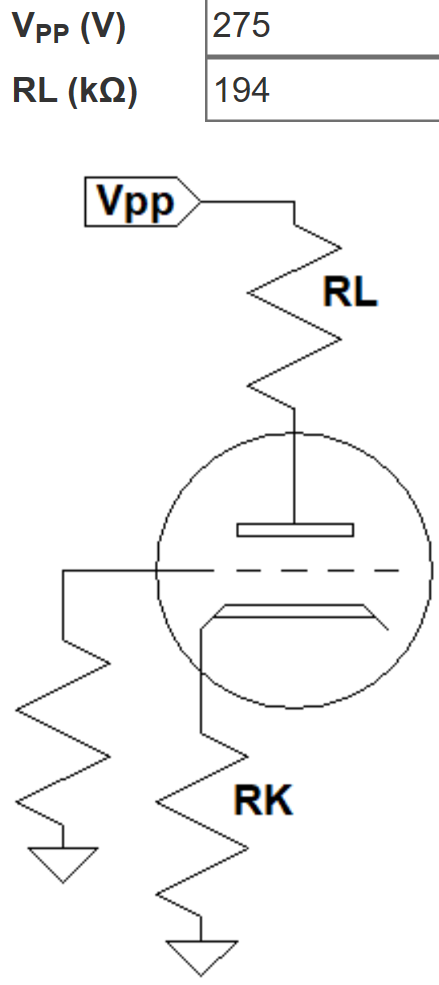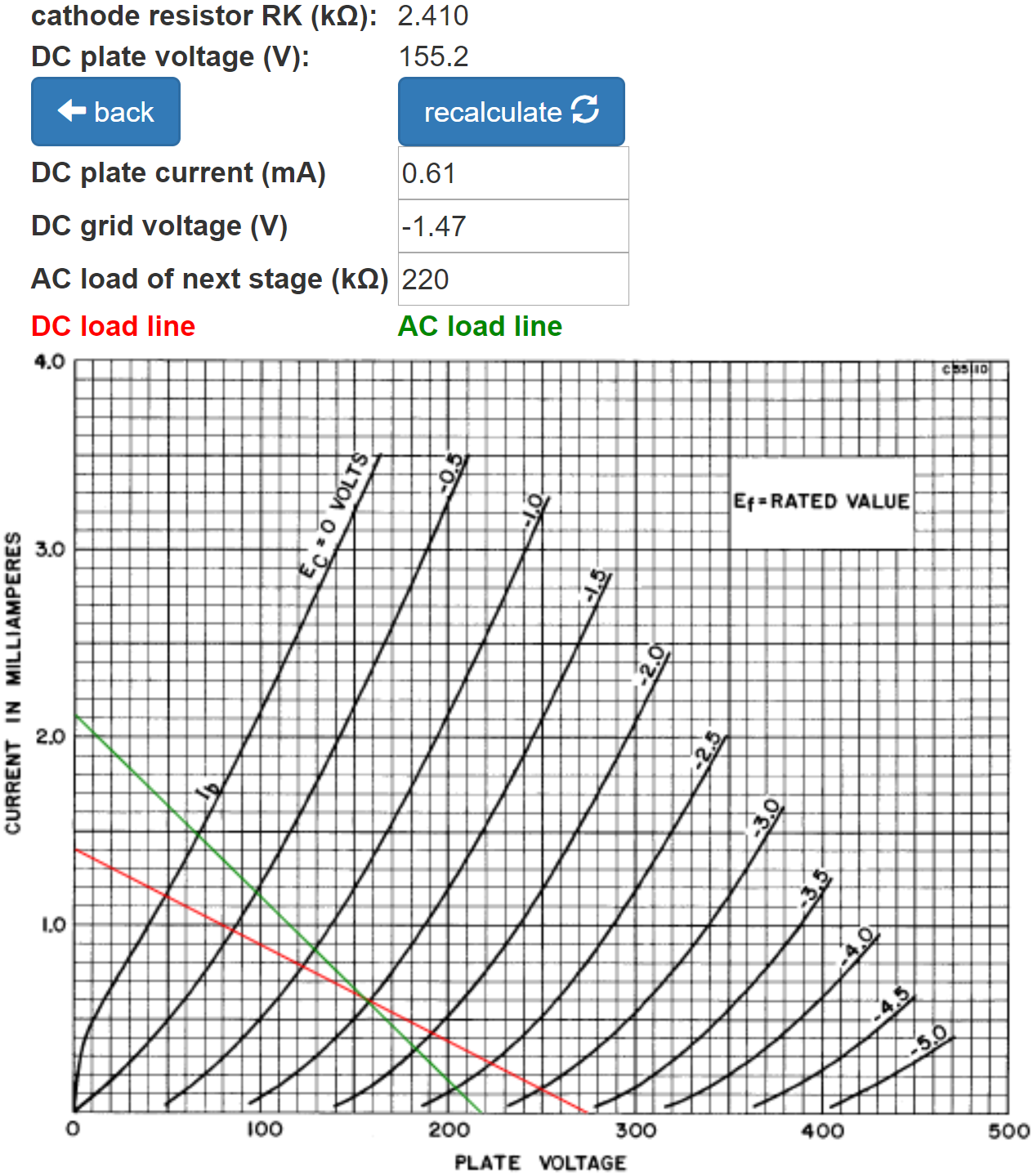The total DC plate supply current for two triodes is double the amount shown: about 1.2mA.

According to the Long Tailed Pair calculator, The 47kΩ resistor creates a large "tail" to balance the inverted and non-inverted gains.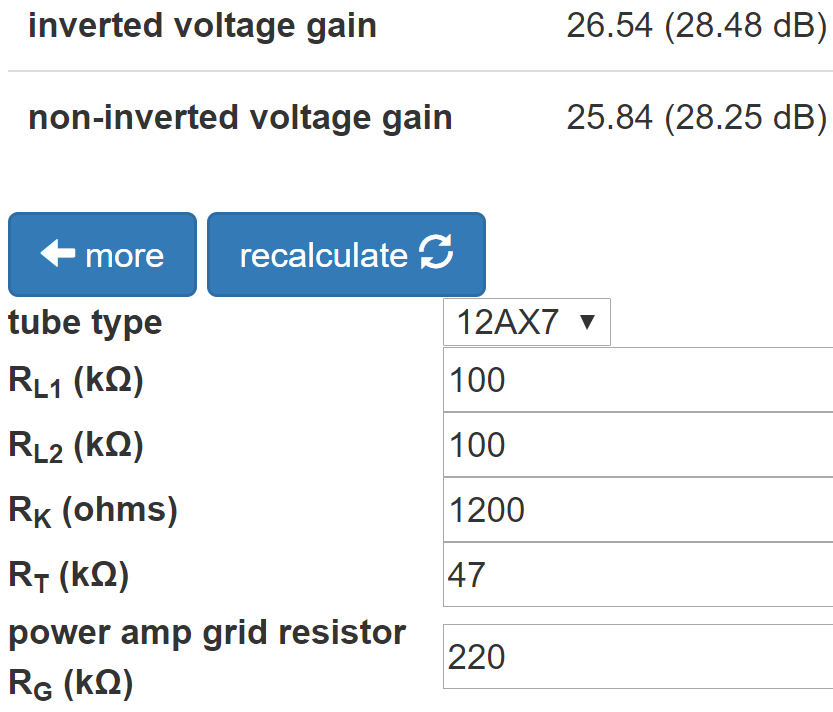When the tail is large enough, reducing the value of the plate load resistor for the inverted output is unnecessary.

The Phase Inverter Bass Response calculator shows that the large 0.1μF coupling capacitors create a flat response.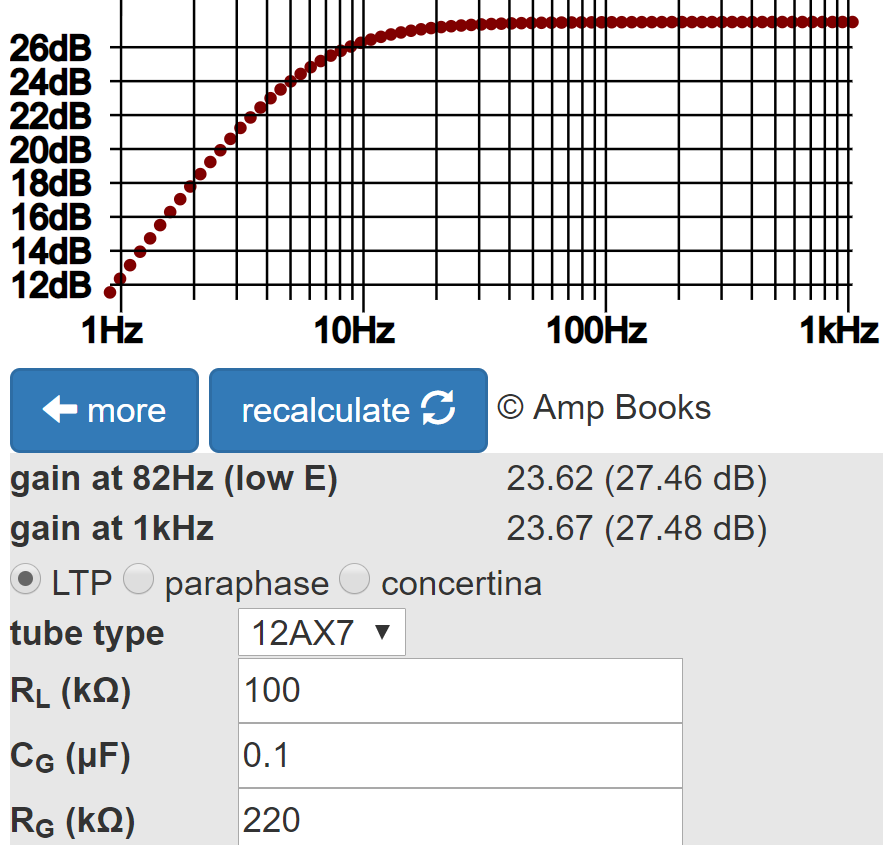Attenuation at 82Hz (low E on a guitar with standard tuning) is only a fraction of a decibel.

#### Parallel, Push-Pull Power Amp

The AC30 push-pull power amp includes four EL84 pentodes, two for each phase.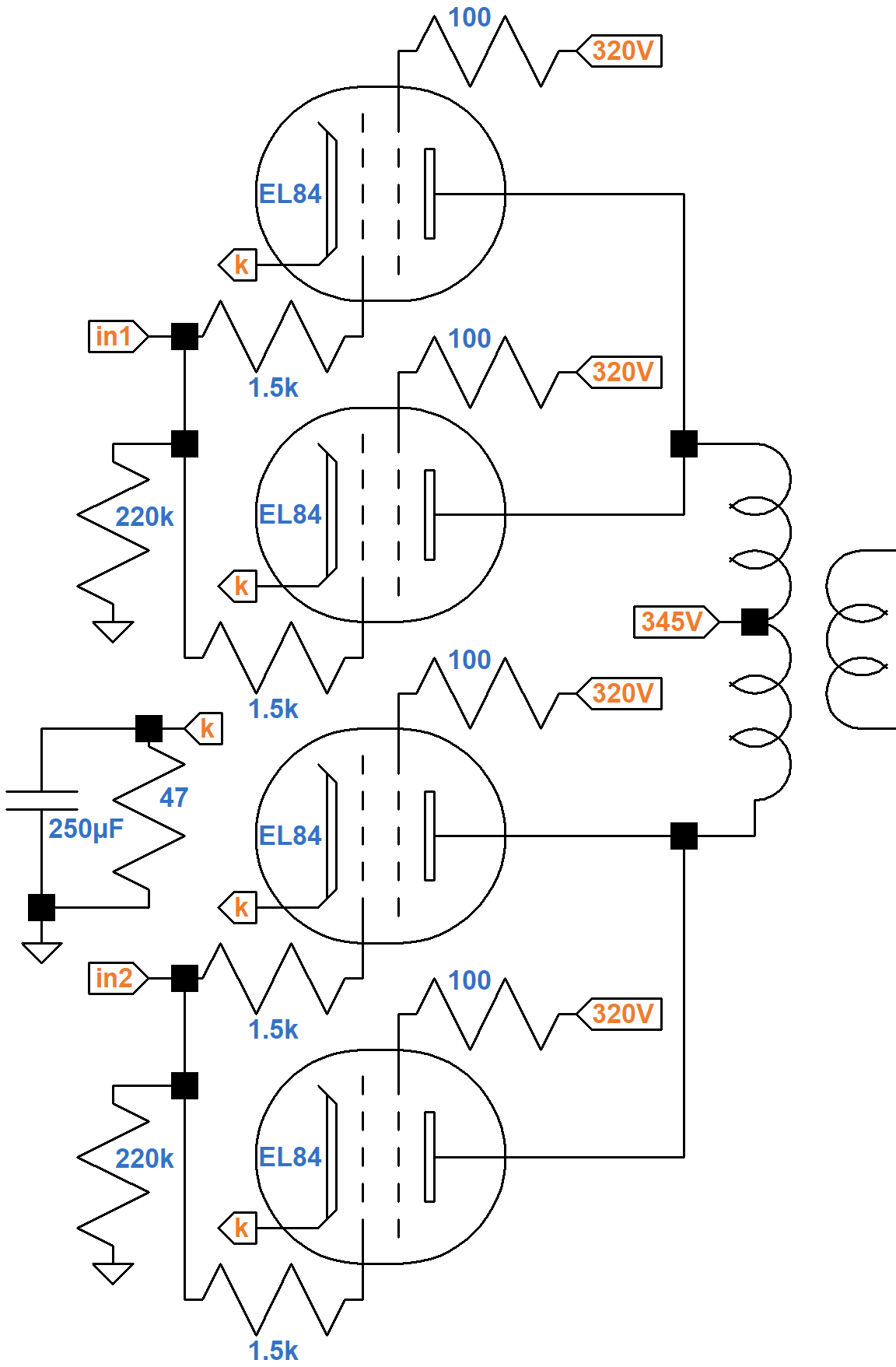The stage is self-biased, so negative DC grid bias is created by raising the cathode voltage relative to ground via the 47Ω cathode resistor. The voltage drop across the screen resistors is negligible at idle, so we can assume the screen voltage is 320V relative to ground.

We can get a close estimate of the DC grid bias by using the triode-connected curves and assuming the plate voltage matches the screen voltage: 320V minus the voltage across the cathode resistor. Screen voltage has a much greater effect on plate current than plate voltage, so the 25V mismatch is relatively insignificant.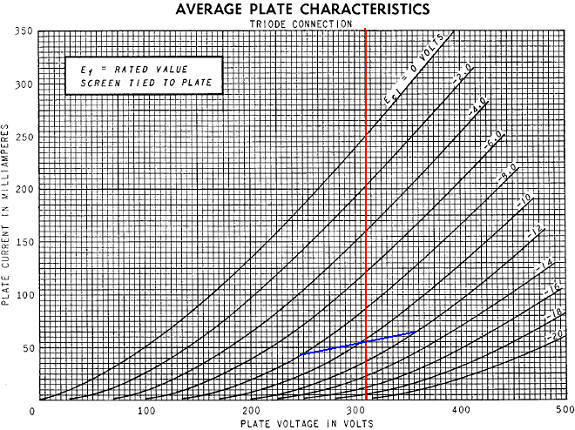The advantage of using this plot is that the "plate current" represents plate and screen current combined, which is exactly what is flowing through the cathodes and the 47Ω cathode resistor to create grid bias. There are four tubes providing cathode current, so the effective cathode resistor value for one tube is quadruple the actual value: 188Ω.

According to Ohm's Law, to create a DC grid bias of -8V, the cathode current needs to be 8V / 188Ω = 43mA. To create a DC grid bias of -10V and -12V, the cathode current needs to be 10V / 188Ω = 53mA and 12V / 188Ω = 64mA. This produces the blue grid line shown on the curves.

For a grid voltage of -10V, the screen and plate voltage is 310V, as shown by the red line. It intersects the blue line very close to -10V grid curve, so for a screen-to-cathode voltage of 310V and a plate-to-cathode voltage of 310V, the cathode-to-ground voltage is 10V and the DC grid bias voltage is -10V. For a plate supply voltage of 345V, the plate-to-cathode voltage is 335V.

To determine the proportion of plate and screen current, we can use the transfer characteristic curves.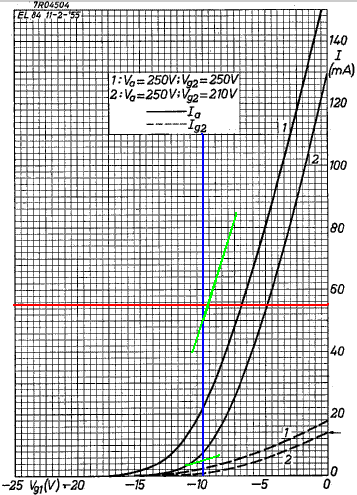The solid black curves depict the plate current versus grid voltage for a screen voltage of 250V (upper curve) and 210V (lower curve). The dashed curves show screen current. The blue line denotes the DC grid bias voltage. The red line is cathode current. Curves for a 310V screen aren't shown but we can imagine that they would be in the vicinity of the green lines.

Where the green lines cross the blue line we observe the plate and screen currents are 50mA and 5mA, respectively, which sum to equal the cathode current.

The cathode resistor carries a lot of current. At idle it dissipates (10V)(55mA) = 550mW per tube for a total of 2.2 watts. Vox specifies a 10-watt rating for the resistor and ensures that it gets plenty of air.

Plate and screen dissipations are (335V)(50mA) = 17W and (310V)(5mA) = 1.6W, respectively. The EL84 limits are 12 watts and 2 watts, respectively, so the AC30 actually exceeds the plate dissipation limit by about 40 percent.

Here are the plate characteristic curves for a screen voltage of 300V, which is close enough to 310V to be used to examine the implications of output transformer primary impedance.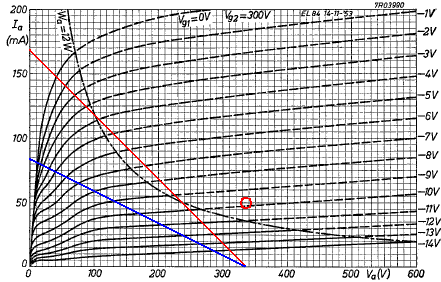The DC operating point is represented by the red circle. It is above the 12W plate dissipation limit, so unlike a typical 6L6 or EL84 Class AB amp, the tubes run fairly hot at idle. At full power one tube grid reaches zero grid volts as the other reaches -20V, putting it into cutoff, so the amp is not pure Class A, although it is certainly closer to Class A than a Plexi. At substanstially less than full power, the power amp can operate as a Class A amp, as can any Class AB amp if the power level is low enough. For the AC30, for example, if the grid swings by 4V peak, i.e. from -6V to -14V, all tubes are above cutoff for a complete cycle.

The AC30 output transformer is 4kΩ plate-to-plate, but there are two tubes in parallel per side, creating an effective 8kΩ plate-to-plate per pair of tubes in push-pull. The impedance creates 2kΩ for the conducting tube, which is depicted by the red line. The blue line shows the effect doubling the effective impedance to 4kΩ. This causes the load line to intersect the 0V grid curve well below the knee, which reduces maximum power and increases screen current at full power.

From the load line we see that a full-power sinewave input signal causes the plate voltage to swing from 335V, when the net plate current through the transformer primary is zero, to 40V where the load line intersects the 0V grid curve. The total swing is 295V. The net plate current swings from zero to a maximum of 147mA. The power output is therefore (335V)(147mA)/2 = 25W measured at the transformer primary.

#### References

1Dave Hunter, Amped, (Minneapolis: Voyageur Press, 2012), p. 78.

2Richard Kuehnel, Guitar Amplifier Electronics: Circuit Simulation, (Seattle: Amp Books, 2019).

3Richard Kuehnel, Guitar Amplifier Electronics: Basic Theory, (Seattle: Amp Books, 2018).New! A deep dive into the electronic design of the ultimate high-gain rig-of-death!From system design concepts to individual stage operation, an all-new examination of Bassman electronics.From "woody" to narrow panel to brownface to blackface Reverb, an in-depth exploration of Fender Deluxe circuitry.Master the basics of preamp, power amp, and power supply design.Design your amp using a structured, professional methodology.Know it works by measuring performance at every point in the amplifier.